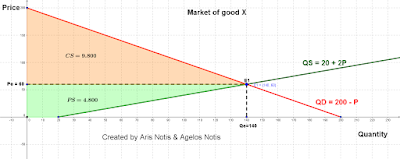## Sunday, 26 March 2017

### Equilibrium

The domestic market of good X is described by the equations: QD = 200 - P & Qs = 20 + 2P. Where QD & Qs is the quantity demanded and supplied in kg respectively and P is the price of the good in US dollars (\$). Calculate the price which consumers and producers are willing and able to pay and receive for the good X respectively.

The price equilibrium in the market of good X is where the demand of goods matches supply. It is calculated by the formula: QD = Qs or 200 - P = 20 + 2P or 3P = 180 or Pe = \$60. Thus, the quantity equilibrium is Qe = 200 - 60 = 140 units of product or Qe = 20 +  (2 x 60) = 140 units of product.
Total Surplus = Consumer Surplus + Producer Surplus = 9800 + 4800 = \$14600.